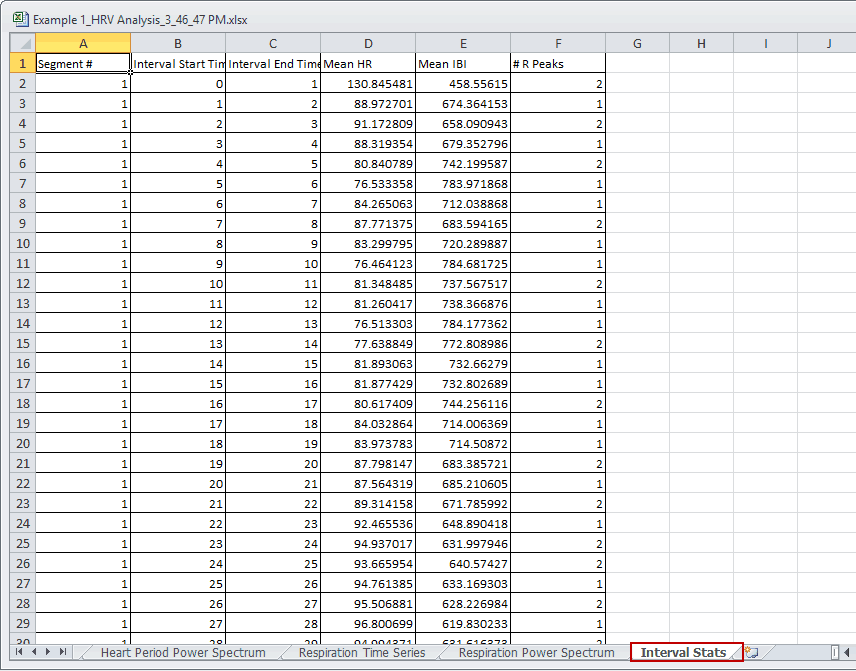# KB0068: Second-by-Second Heart Rate Using Intervals

It is possible to determine second-by-second heart rate values using Interval mode in the Heart Rate Variability analysis application.  Interval mode can be enabled by navigating to the HRV Calibration Settings tab and changing the Calculation Method to Interval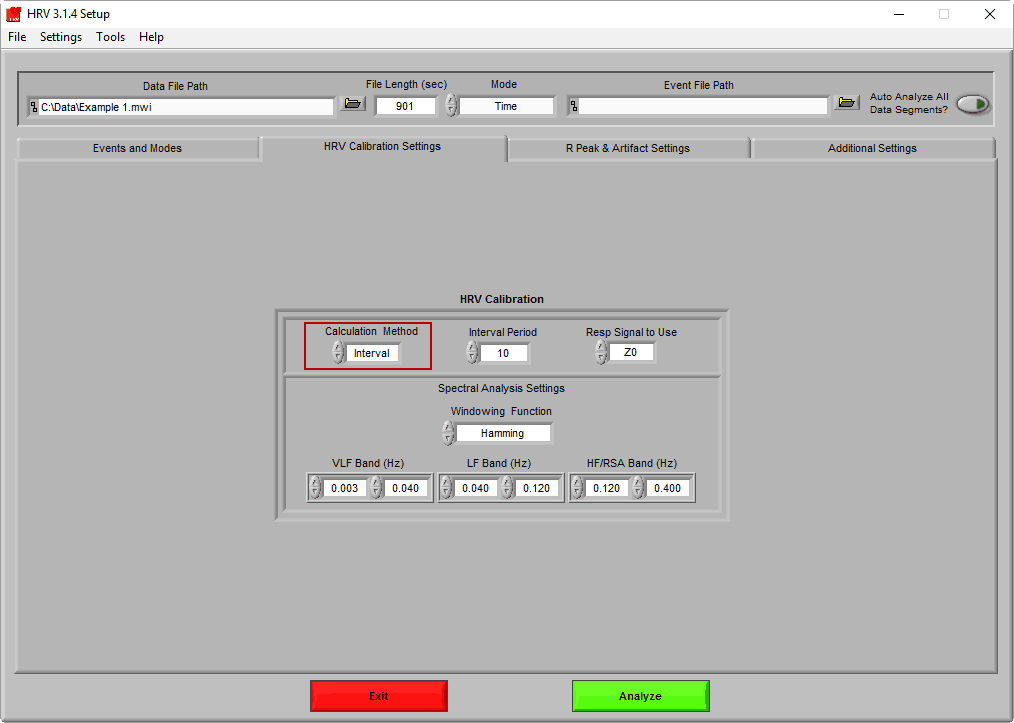Interval mode allows you to split a given segment into smaller “intervals”, for which select statistics are calculated in addition to the statistics for the entire segment. When Interval mode is selected, the Interval Period parameter is enabled. Interval period is the number of seconds which make up a single interval.

To calculate second-by-second heart rate, change Interval Period to 1.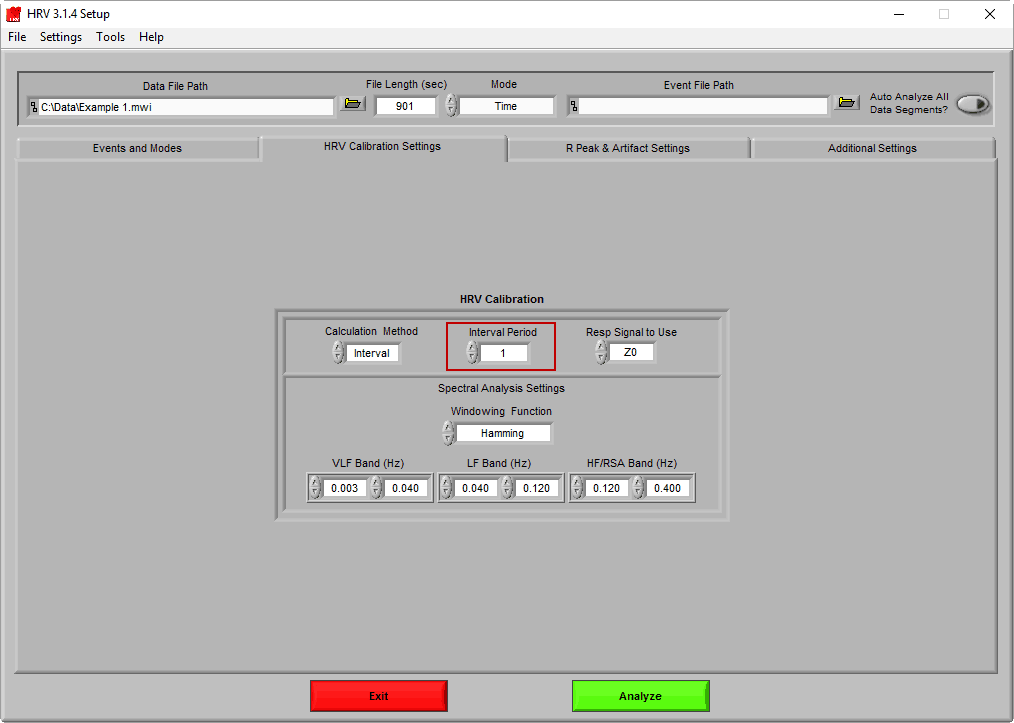Press Analyze to proceed to the analysis screen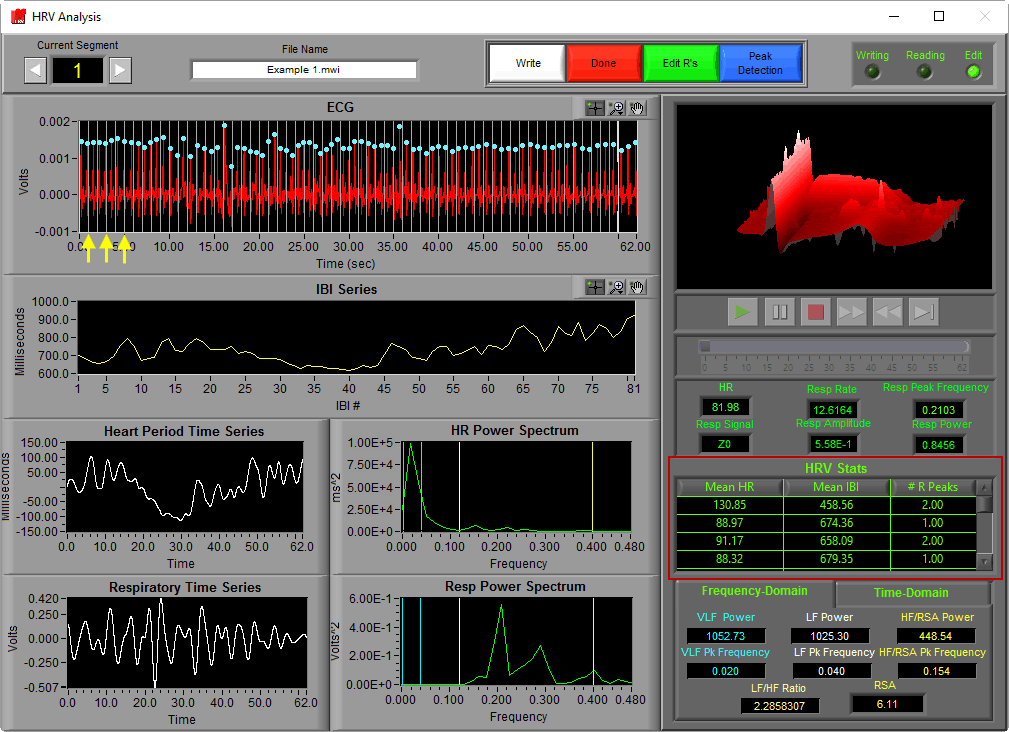The thin grey vertical cursors in the ECG plot define the intervals in the segment. For each of these intervals, there are 3 statistics calculated in the HRV Stats table:

• Mean Heart Rate
• Mean IBI
• # R Peaks

To effectively calculate second-by-second heart rate, where some intervals could have no actual detected R peaks in them, the Graham method of weighted heart periods is utilized.

For this method to work, the percentage of a heart period which lies within a given interval needs to be determined. Notice that even though the segment time for this example was set to 60 seconds, there are actually 62 seconds being displayed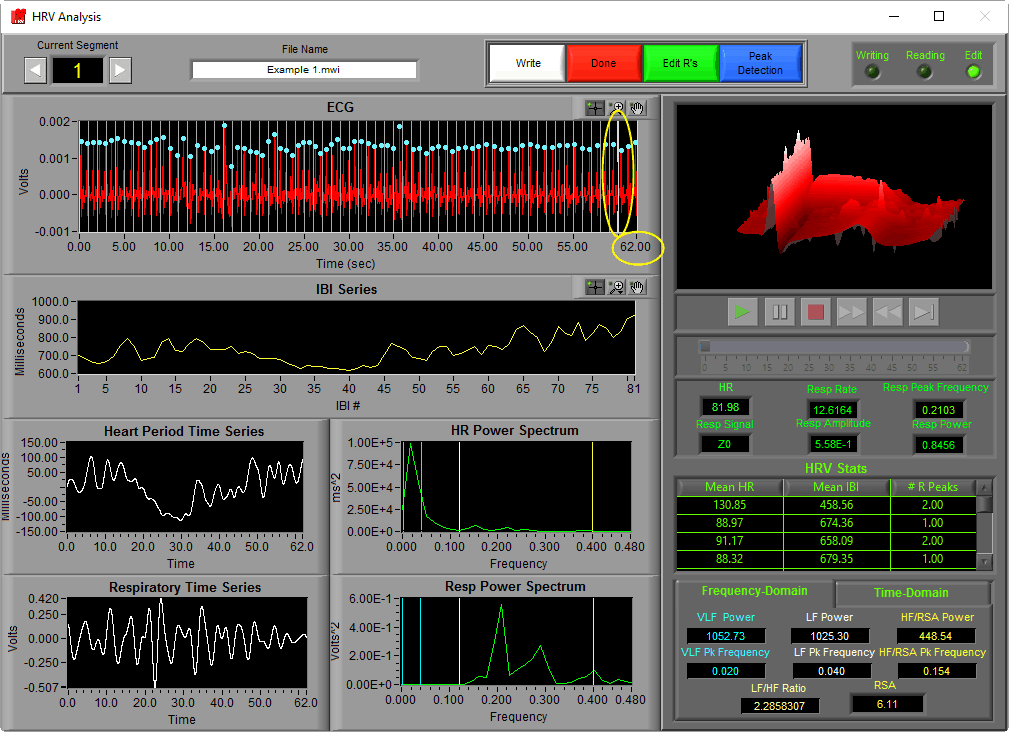In order to calculate the statistics for the first/last intervals in the segment, we need to know information about the beats that immediately precede or follow them.

The actual segment for which all statistics are calculated is framed by the thick grey cursors, and all data on either side is only used for interval-based stats. This additional data can (and should) be edited for correctness to properly calculate second-by-second heart rate.

Note:  The first and last intervals in a data file do not have sufficient information to accurately calculate heart rate

All interval statistics are written into the Excel output file for additional processing.